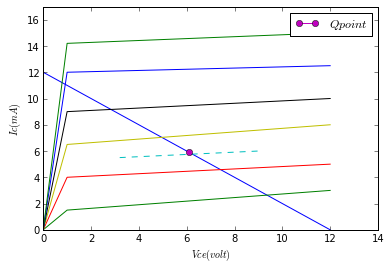# Chapter 5: Transistors¶

### Example 5.1 Page No 134¶

In :
alpha=0.98                       #alpha(dc),  current gain
Ico=1*10**(-6)               #Ampere, collector leakage current
Ie=1*10**(-3)                 # Ampere, emitter current

#Calculation
Ic=alpha*Ie+Ico             #Collector Current
Ib=Ie-Ic                          #Base Current
#result
print " The Collector Current is Ic=  ",Ic*1000,"mA"
print " The Base Current is Ib= ",Ib*10**6,"microA"

 The Collector Current is Ic=   0.981 mA
The Base Current is Ib=  19.0 microA


### Example 5.2 Page No 141¶

In :
dIe=(0.7-0.3)*10**(-3)                        #A, change in emitter current
dVeb=(0.7-0.62)                                  #V, change in emitter base voltage
#Calculation
ri=dVeb/dIe                                      #Dynamic Input Resistance at Vcb= -10 V
#Result
print " The Dynamic Input Resistance is ri=  ",ri,"ohm"

 The Dynamic Input Resistance is ri=   200.0 ohm


### Example 5.3 Page No 144¶

In [ ]:
dIe=1*10**(-3)                  #A, change in emitter current
dIc=0.99*10**(-3)             #A, change in the collector current

#Calculation
hfb=dIc/dIe                       #Short Circuit Current Gain
#Result
print "The Short Circuit Current Gain is alpha or hfb= ",hfb


### Example 5.4 Page No 147¶

In :
dIe=1*10**(-3)                           #A, change in emitter current
dIc=0.995*10**(-3)                    #A, change in collector current

#Calculation
alpha=dIc/dIe                              #Common Base Short Circuit Current Gain
#Result
print " The Common Base Short Circuit Current Gain is alpha= ",alpha

#(b)
beeta=alpha/(1-alpha)                  #Common Emitter Short Circuit Current Gain
# Result
print " The Common Emitter Short Circuit Current Gain is beeta= ",beeta

 The Common Base Short Circuit Current Gain is alpha=  0.995
The Common Emitter Short Circuit Current Gain is beeta=  199.0


### Example 5.5 Page No 147¶

In :
Beeta=100.0                            #dc current gain

#Calculation
Alpha=Beeta/(Beeta+1)    #DC Current Gain in Common Base Configuration
# Result
print " The DC Current Gain in Common Base Configuration is Alpha= ",round(Alpha,2)

 The DC Current Gain in Common Base Configuration is Alpha=  0.99


### Example 5.6 Page No 150¶

In :
Vce=10                                       #V, collector emitter voltage
Ib=30*10**(-6)                        #A, base current

#Calculation from Given Output Characteristics at Ib = 30uA
dVce=(12.5-7.5)                      #V, change in collector emitter voltage
dic=(3.7-3.5)*10**(-3)           #A, change in collector current
Ic=3.6*10**(-3)                      #A, collector current at operating point
ro=dVce/dic                            # Dynamic Output Resistance
Beeta_dc=Ic/Ib                      # DC Current Gain
Beeta_ac=((4.7-3.6)*10**(-3))/((40-30)*10**(-6))                #AC Current Gain, From Graph, Bac=delta(ic)/delta(ib) for given Vce

# Result
print "Dynamic Output Resistance ,ro = ",ro/10**(3),"kohm"
print " DC Current Gain ,Bdc = ",Beeta_dc
print " AC Current Gain ,Bac = ",Beeta_ac

Dynamic Output Resistance ,ro =  25.0 kohm
DC Current Gain ,Bdc =  120.0
AC Current Gain ,Bac =  110.0


### Example 5.7 Page No 159¶

In :

Vcc=12                                #V, collector bias juncyion voltage
Rc=1000.0                           #Ohms, collector resistance
Vbb=10.7                            #V.  base bias junction voltage
Rb=200000.0                      #Ohms, base resistance
Vbe=0.7                              #V, base emitter voltage

#Calculation
Ib=(Vbb-Vbe)/Rb             # base current
#Value of Ib comes out to be 50uA. A dotted Curve is drawn for
#Ib=40uA and Ib=60uA.   At the Point of Intersection:
Vce=6                                 #V, collector emitter voltage
Ic=6*10**(-3)                   #A, collector current
# Result
print " Q point:  Ib = ",Ib/10**(-6),"microA"
print " Vce = ",Vce,"V"
print " Ic =  ",Ic/10**(-3),"mA"
#Plot

#DC Load LIne  AT Ib=50 microA
Vce1=[0,12]
Ic1=[12,0]
a1=plot(Vce1,Ic1)
xlim(0,14)
ylim(0,17)

# AT Ib=20 microA
Vce2=[0,1,12]
Ic2=[0,1.5,3]
a2=plot(Vce2,Ic2)

# AT Ib=40 microA
Vce3=[0,1,12]
Ic3=[0,4,5]
a3=plot(Vce3,Ic3)

#At IB=50
Vcex=[3.2,9]
Icx=[5.5,6]
ax=plot(Vcex,Icx,linestyle='--')
qx=plot(6.1,5.9,marker='o',label='$Q point$')
legend()

# AT Ib=60 microA
Vce4=[0,1,12]
Ic4=[0,6.5,8]
a4=plot(Vce4,Ic4)

# AT Ib=80 microA
Vce5=[0,1,12]
Ic5=[0,9,10]
a5=plot(Vce5,Ic5)

# AT Ib=100 microA
Vce6=[0,1,12]
Ic6=[0,12,12.5]
a6=plot(Vce6,Ic6)

# AT Ib=120 microA
Vce7=[0,1,12]
Ic7=[0,14.2,15]
a7=plot(Vce7,Ic7)
xlabel("$Vce(volt)$")
ylabel("$Ic(mA)$")
show(a1)
show(ax)
show(qx)
show(a2)
show(a3)
show(a4)
show(a5)
show(a6)
show(a7)

 Q point:  Ib =  50.0 microA
Vce =  6 V
Ic =   6.0 mA### Example 5.8 Page No 173¶

In :
u=80.0                                      # Amplification Factor
gm=200*10**(-6)                   # S, Transconductance

#Calculation
rd=u/gm                                #Dynamic Drain Resistance
# Result
print " The Dynamic Drain Resistance of JFET is rd= ",rd/10**(3),"kohm"

 The Dynamic Drain Resistance of JFET is rd=  400.0 kohm